# How to Remove Array Elements in Java

Published on August 3, 2022By Jayant Verma
Developer and author at DigitalOcean.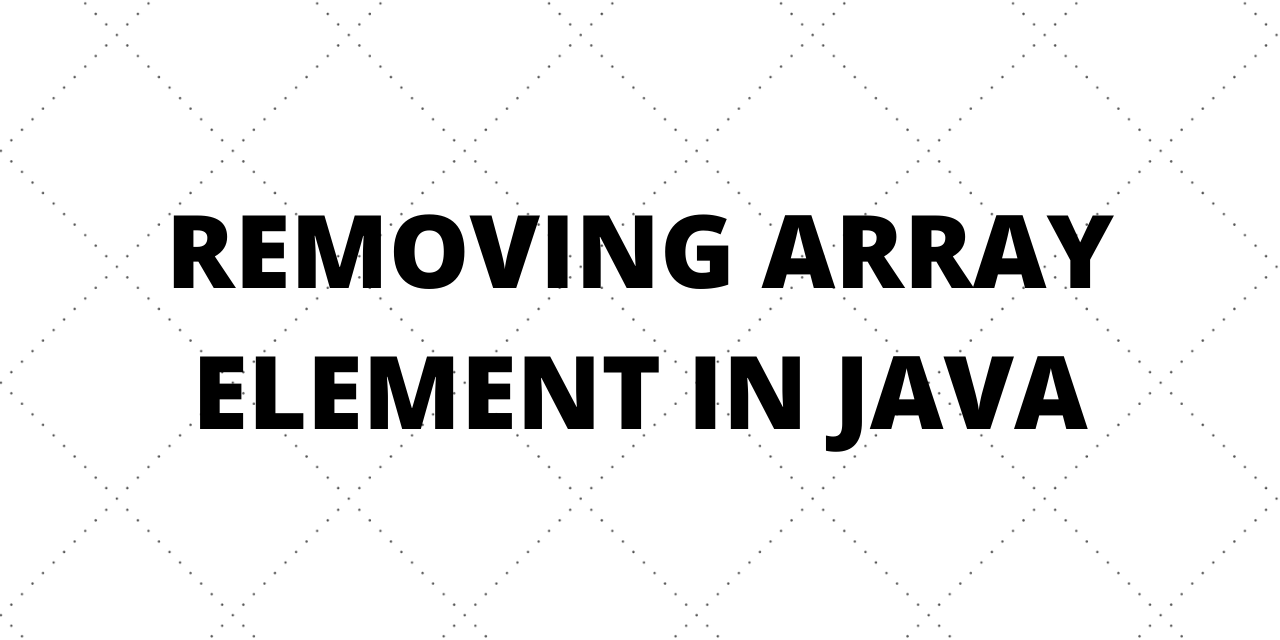While we believe that this content benefits our community, we have not yet thoroughly reviewed it. If you have any suggestions for improvements, please let us know by clicking the “report an issue“ button at the bottom of the tutorial.

When we create an array in Java, we specify its data type and size. This is used by JVM to allocates the necessary memory for array elements. There are no specific methods to remove elements from the array.

## 1. Removing an element from Array using for loop

This method requires the creation of a new array. We can use for loop to populate the new array without the element we want to remove.

``````package com.journaldev.java;

import java.util.Arrays;

public class Main {

public static void main(String[] args) {
int[] arr = new int[]{1,2,3,4,5};
int[] arr_new = new int[arr.length-1];
int j=3;
for(int i=0, k=0;i<arr.length;i++){
if(i!=j){
arr_new[k]=arr[i];
k++;
}
}
System.out.println("Before deletion :" + Arrays.toString(arr));
System.out.println("After deletion :" + Arrays.toString(arr_new));

}
}
``````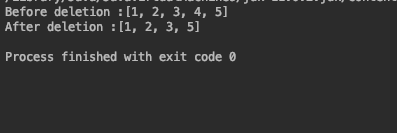The code removes the element at index 3. This method simply copies all the elements except the one at index 3 to a new array.

## 2. Deleting an array element by its value

Unlike the previous case, this code will delete the element based on its value. This will not work with duplicates since the size of the array after deletion has to be known.

``````package com.journaldev.java;
import java.util.Arrays;

public class Main {

public static void main(String[] args) {
int[] arr = new int[]{1,2,3,4,5};
int[] arr_new = new int[arr.length-1];
int j=3;
for(int i=0, k=0;i<arr.length;i++){
if(arr[i]!=j){
arr_new[k]=arr[i];
k++;
}
}
System.out.println("Before deletion :" + Arrays.toString(arr));
System.out.println("After deletion :" + Arrays.toString(arr_new));

}
}
``````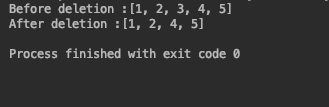The only difference between this and the previous case is `arr[i]!=j` in the if condition in place of `i!=j`.

## 3. Deleting element by its value when the array contains duplicates

Performing deletion based on the value in case of duplicates requires using ArrayList. Since ArrayList doesn’t require the size to be now in advance, it allows us to expand dynamically.

``````package com.journaldev.java;

import java.util.ArrayList;
import java.util.Arrays;

public class Main {

public static void main(String[] args) {
int[] arr = new int[]{1,3,3,4,5};
ArrayList<Integer> arr_new = new ArrayList<>();
int j=3;
for(int i=0;i<arr.length;i++){
if(arr[i]!=j){

}
}
System.out.println("Before deletion :" + Arrays.toString(arr));
System.out.println("After deletion :" +arr_new);

}
}
``````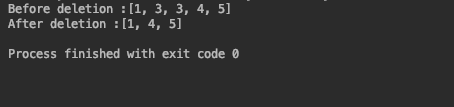## 4. Shifting elements in the same array

This method involves shifting the elements in the same array. Shifting of elements replaces the element to be deleted by the element at the next index.

``````package com.journaldev.java;
import java.util.Arrays;
public class Main {

public static void main(String[] args) {
int[] arr = new int[]{1,3,3,4,5};
int j=3;
System.out.println("Before deletion :" + Arrays.toString(arr));
int count =0;
for(int i = 0; i < arr.length; i++){
if(arr[i] == j){
count++;

// shifting elements
for(int k = i; k < arr.length - 1; k++){
arr[k] = arr[k+1];
}
i--;
// break;
}
}

System.out.print("After Deletion :" );
for(int i = 0; i < arr.length-count; i++){
System.out.print(" " + arr[i]);
}
System.out.println();
System.out.println("Whole array :" + Arrays.toString(arr));

}
}
``````

Count variable indicates the number of elements deleted. This variable is essential to keep a track of index till which the array should be printed. This method takes care of duplicates as well.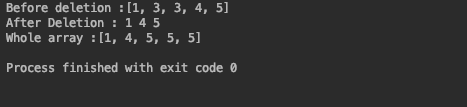## 5. Deleting elements from ArrayList

ArrayList is backed by arrays. The deletion of an element in the ArrayList is straight forward. It requires one simple call to an inbuilt function.

``````package com.journaldev.java;
import java.util.ArrayList;
import java.util.Arrays;
public class Main {

public static void main(String[] args) {
int[] arr = new int[]{1,3,3,4,5};
ArrayList<Integer> arr_new = new ArrayList<Integer>();
for (int i : arr)
{
}
arr_new.remove(3);
System.out.println("Before deletion :" + Arrays.toString(arr));
System.out.println("After Deletion:" + arr_new);
}
}
``````

A call to the `remove(i)` function removes the element at index i. Deletion in ArrayLists is relatively easier as compared to Arrays.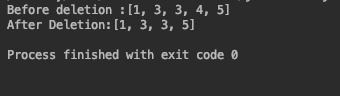## Conclusion

We saw some examples of deleting elements in an array using different methods. The difference between the deletion of an element in an Array and an ArrayList is clearly evident. If deletion is to be performed again and again then ArrayList should be used to benefit from its inbuilt functions. Same is the case with the addition of elements to an array. ArrayList due to its dynamic nature provides easier and less cumbersome ways to alter an array.Developer and author at DigitalOcean.

#### Still looking for an answer?

I was deleting an array element by shifting , similar to what you have done in method 4. Came here to see if I could do something to get rid of the duplicate elements that remain at the end of the array. Wanted to do this without copying to new array.

- Meena